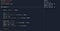# Passing Pointers in a Function

Those who are new to c,c++ programming might find it challenging to understand some of the concepts.

One such concept is of Pointer. Pointer is a variable whose value is the address of another variable.

Example:- We created a variable i of type int having a value 10. Then we printed the address of i using the operator “&i” .

• From the output we can see the address of i = 0x7ffddedd24c, this is the memory location/address assigned to 10.
• Then we assigned the address to the pointer p and printed out the value of p
• we can see in the output that the value of p is an address i.e 0x7ffddedd24c , which is same as that of address of 10 or i.
• To find which value is the address pointing to we use dereference operator , that comes out to be 10.

# Data Types of Pointer:-

`int *p;          /* pointer to an integer */double *p;      /* pointer to a double */float *p;      /* pointer to a float */char *h       /* pointer to a character */ `

The actual data type of the value of all pointers, whether integer, float, character .etc. is the same, a long hexadecimal number that represents a memory address.

The only difference between pointers of different data types is the data type of the variable or constant that the pointer points to.size of pointer is always same no matter which datatype it’s pointing towards.

Also size of the pointer is same in every case.

Now we know that what pointer is and we will move to the concept most find a little difficult to understand in the beginning i.e. Passing pointer in a function.

# Passing Pointer in the function:-

Passing of pointer in function is very different then passing a normal variable .
We will see it by a simple example.

What were we trying to achieve through this code was to increase the value that the pointer is pointing to. i.e. increase the value of i form 10 ->11.

So we created two function increment_1 and increment_2 function and observed they behave differently increment_1 was unable to increase the value at i , while increment_2 was able to increase the value. But the code appears to be right in increment_1, what went wrong?

This happen as when the pointer is passed through the function a temporary pointer is created and that gets destroyed when the function calls ends.

1. Increment_1:- In this case the new pointer is created at a new location let say address = 800 , so when p=p+1 happens it will increase the pointer location from 800 to 808 , which will point to a different location and will contain so garbage value.

But this pointer will get destroyed and thus no change in main function value occurs. Thus *p = 10 after increment_1() function call ends.

2. Increment_2():- Now in case of increment_2 again a new temp pointer is created at location address = 800 . But this time since the new pointer contains the address of actual main pointer thus when we do *P it start again pointing to the main pointer , thus now if we do (*p)++

It will increase the value that the main pointer is pointing towards i.e 10
so 10++ = 11. Hence we see an increased value this time too.

Hope you now get an idea what things behave differently in case of pointer.